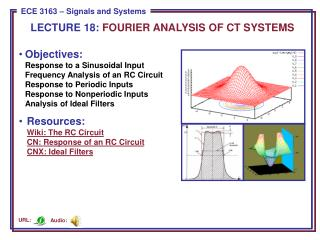Download PresentationLECTURE 18: FOURIER ANALYSIS OF CT SYSTEMS

# LECTURE 18: FOURIER ANALYSIS OF CT SYSTEMS - PowerPoint PPT PresentationDownload Presentation## LECTURE 18: FOURIER ANALYSIS OF CT SYSTEMS

- - - - - - - - - - - - - - - - - - - - - - - - - - - E N D - - - - - - - - - - - - - - - - - - - - - - - - - - -
##### Presentation Transcript

1. LECTURE 18: FOURIER ANALYSIS OF CT SYSTEMS • Objectives:Response to a Sinusoidal InputFrequency Analysis of an RC CircuitResponse to Periodic InputsResponse to Nonperiodic InputsAnalysis of Ideal Filters • Resources:Wiki: The RC CircuitCN: Response of an RC CircuitCNX: Ideal Filters Audio: URL:

2. Response of an LTI System to a Sinusoid • Consider an LTI CT system with impulse response h(t): • We will assume that the Fourier transform of h(t) exists: • The output can be computed using our Fourier transform properties: • Suppose the input is a sinusoid: • Using properties of the Fourier transform, we can compute the output:

3. Example: RC Circuit • Using our FT properties: • Compute the frequency response: • RC = 0.001; • W=0:50:5000; • H=(1/RC)./(j*w+1/RC); • magH=abs(H); • angH=180*angle(H)/pi;

4. Example: RC Circuit (Cont.) • We can compute the output for RC=0.001 and 0=1000 rad/sec: • We can compute the output for RC=0.001 and 0=3000 rad/sec: • Hence the circuit acts as a lowpass filter. Note the phase is not linear. • If the input was the sum of two sinewaves: • describe the output.

5. Response To Periodic Inputs • We can extend our example to all periodic signals using the Fourier series: • The output of an LTI system is: • We can write the Fourier series for the output as: • It is important to observe that since the spectrum of a periodic signal is a line spectrum, the output spectrum is simply a weighted version of the input, where the weights are found by sampling of the frequency response of the LTI system at multiples of the fundamental frequency, 0.

6. Example: Rectangular Pulse Train and an RC Circuit • Recall the Fourier series fora periodic rectangular pulse: • Also recall the system response was: • The output can be easily written as:

7. Example: Rectangular Pulse Train (Cont.) • We can write a similar expression for the output: 1/RC = 1 • We can observe the implications of lowpass filtering this signal. • What aspects of the input signal give rise to high frequency components? • What are the implications of increasing 1/RC in the circuit? • Why are the pulses increasingly rounded for lower values of 1/RC? • What causes the oscillations in the signal as 1/RC is increased? 1/RC = 10 1/RC = 100

8. Response to Nonperiodic Inputs • We can recover the output in the time domain using the inverse transform: • These integrals are often hard to compute, so we try to circumvent them using transform tables and combinations of transform properties. • Consider the response of our RC circuit to a single pulse: • MATLAB code for the frequency response: • RC=1; • w=-40:.3:40; • X=2*sin(w/2)./w; • H=(1/RC)./(j*w+1/RC); • Y=X.*H; • magY=abs(Y);

9. Response to Nonperiodic Inputs (Cont.) • We can recover the output using the inverse Fourier transform: • syms X H Y y w • X = 2*sin(w/2)./w; • H=(1/RC)./(j*w+1/RC); • Y=X.*H; • Y=ifourier(Y); • ezplot(y,[-1 5]); • axis([-1 5 0 1.5]) 1/RC = 1 1/RC = 1 1/RC = 10 1/RC = 10

10. Ideal Filters • The process of rejecting particular frequencies or a range of frequencies is called filtering. A system that has this characteristic is called a filter. • An ideal filter is a filter whose frequency response goes exactly to zero for some frequencies and whose magnitude response is exactly one for other ranges of frequencies. • To avoid phase distortion in the filtering process, an ideal filter should have a linear phase characteristic. Why? • We will see this “ideal” response has some important implications for the impulse response of the filter. • Highpass • Lowpass • Bandstop • Bandpass

11. Ideal Linear Phase Lowpass Filter • Consider the ideal lowpass filterwith frequency response: • Using the Fourier transform pairfor a rectangular pulse, and applyingthe time-shift property: • Is this filter causal? • The frequency response of an idealbandpass filter can be similarly defined: • Will this filter be physically realizable?Why? • PhaseResponse • ImpulseResponse

12. Summary • Showed that the response of a linear LTI system to a sinusoid is a sinusoid at the same frequency with a different amplitude and phase. • Demonstrated how to compute the change in amplitude and phase using the system’s Fourier transform. • Demonstrated this for a simple RC circuit. • Generalized this to periodic and nonperiodic signals. • Worked examples involving a periodic pulse train and a single pulse. • Introduced the concept of an ideal filter and discussed several types of ideal filters. • Noted that the ideal filter is a noncausal system and is not physically realizable. However, there are many ways to approximate ideal filters, and that is a topic known as filter design.